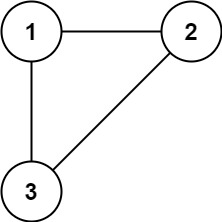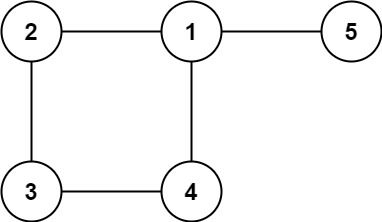0684. 冗余连接

# 0684. 冗余连接#

• 标签：深度优先搜索、广度优先搜索、并查集、图
• 难度：中等

## 题目大意 #

• $n == edges.length$。
• $3 \le n \le 1000$。
• $edges[i].length == 2$。
• $1 \le ai < bi \le edges.length$。
• $ai ≠ bi$。
• $edges$ 中无重复元素。
• 给定的图是连通的。1 2  输入: edges = [[1,2], [1,3], [2,3]] 输出: [2,3]1 2  输入: edges = [[1,2], [2,3], [3,4], [1,4], [1,5]] 输出: [1,4] 

## 解题思路 #

### 思路 1：并查集 #

1. 从前向后遍历每一条边。
2. 如果边的两个节点不在同一个集合，就加入到一个集合（链接到同一个根节点）。
3. 如果边的节点已经出现在同一个集合里，说明边的两个节点已经连在一起了，再加入这条边一定会出现环，则这条边就是所求答案。

### 思路 1：代码 #

  1 2 3 4 5 6 7 8 9 10 11 12 13 14 15 16 17 18 19 20 21 22 23 24 25 26 27 28 29 30  class UnionFind: def __init__(self, n): self.parent = [i for i in range(n)] def find(self, x): while x != self.parent[x]: self.parent[x] = self.parent[self.parent[x]] x = self.parent[x] return x def union(self, x, y): root_x = self.find(x) root_y = self.find(y) self.parent[root_x] = root_y def is_connected(self, x, y): return self.find(x) == self.find(y) class Solution: def findRedundantConnection(self, edges: List[List[int]]) -> List[int]: size = len(edges) union_find = UnionFind(size + 1) for edge in edges: if union_find.is_connected(edge, edge): return edge union_find.union(edge, edge) return None 

### 思路 1：复杂度分析 #

• 时间复杂度：$O(n \times \alpha(n))$。其中 $n$ 是图中的节点个数，$\alpha$ 是反 Ackerman 函数。
• 空间复杂度：$O(n)$。